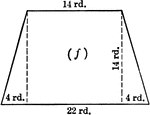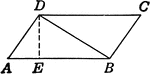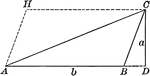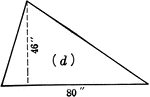Trapezoid With Dimensions

Trapezoid with dimensions labeled. Trapezoid can be used to calculate area.Area of Triangle

Parallelogram illustration to show where the area of a triangle formula comes from.Area of a Triangle

Illustration of triangle ABC with altitude a and base b to be used to demonstrate that the area is 1/2ba.Triangle With Dimensions

Triangle with dimensions labeled. Triangle can be used to calculate area.Triangle With Dimensions

Triangle with dimensions labeled. Triangle can be used to calculate area.